Maths Index
Mathematics

Mathematical Operations

 Integration by Parts Integration by parts is a technique for performing integration by expanding the differential of a product of functions and expressing the original integral in terms of a known integral .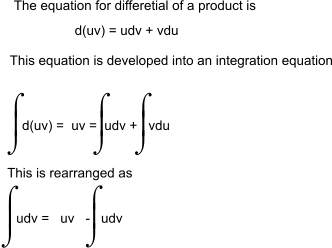A simple example illustrates the method of Integration by parts. The requirement is to integrate the product x.cos(x)dx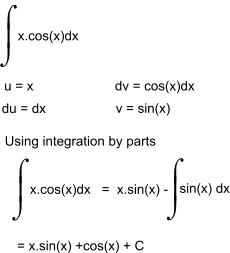Partial Fractions..Partial fractions are also covered on webpage laplace Transform -partial fraction A complicated algebraic fraction can often be reduced to to its equivalent partial fractions such that it allows an integration process to be simplified.    A typical example is shown below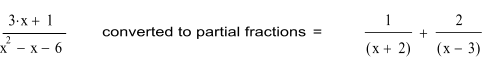Rules for Partial fractions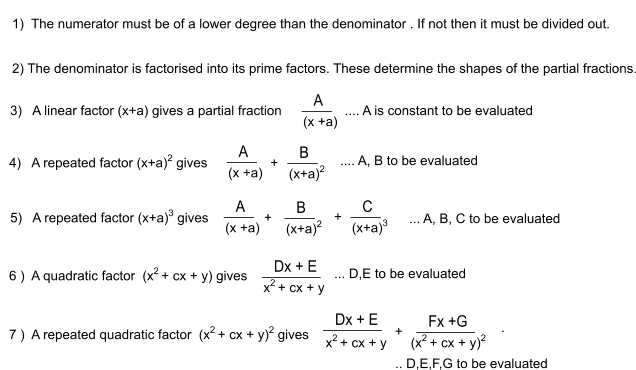A simple example of the application of these rules is as follows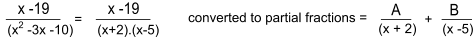The equations are solved for A and B as follows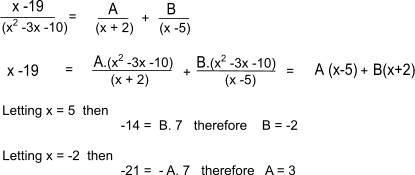A second example is provide below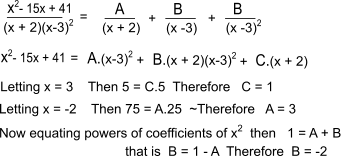Partial Fractions -Cover up rule There are many simple cases where the cover up method can be used . This method only applies if the denominator of the original fraction has non-repeated , linear factors. The method is illustrated by the following example.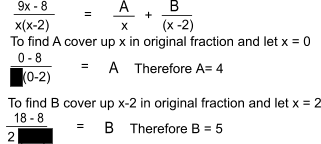Useful Related Links Platonic Realms ...Very Extensive Mathematics Information /formulae. The Maths Pages.. Easy to follow mathematics notes. Scienceworld.wolfram .... Welcome to the best resource of Science and math on the Internet - Probably true Karls Calculus Tutor .... This is an excellent site for learning Calculus
Maths Index
Mathematics